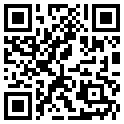Network (GH/s)
Difficulty
Masternodes
Coin Supply (AGU)
BTC PriceaQzzLur2mUzjye5ir6APtVAz2HD7KRvYS3
Total Sent (AGU)Total Received (AGU)Balance (AGU)
7534.7559316111376.635921213841.87998960
Latest Transactions
Amount (AGU)
+ 839.43003120
+ 839.43003120
+ 839.43003120
+ 839.43003120
+ 839.43003120
+ 839.43003120
+ 839.43003120
+ 839.43003120
+ 839.43003120
+ 839.43003120
+ 839.43003120
+ 839.43003120
+ 839.43003120
+ 839.43003120
+ 839.43003120
+ 839.43003120
+ 839.43003120
+ 839.43003120
+ 839.43003120
+ 839.43003120
+ 839.43003120
+ 839.43003120
+ 839.43003120
+ 839.43003120
+ 839.43003120
+ 839.43003120
+ 839.43003120
+ 839.43003120
+ 839.43003120
+ 839.43003120
+ 839.43003120
+ 839.43003120
+ 839.43003120
+ 839.43003120
+ 839.43003120
+ 839.43003120
+ 839.43003120
+ 839.43003120
+ 839.43003120
+ 839.43003120
+ 839.43003120
+ 839.43003120
+ 839.43003120
+ 839.43003120
+ 839.43003120
+ 839.43003120
+ 839.43003120
+ 839.43003120
+ 839.43003120
+ 839.43003120
+ 839.43003120
+ 839.43003120
+ 839.43003120
+ 839.43003120
+ 839.43003120
+ 839.43003120
+ 839.43003120
+ 839.43003120
+ 839.43003120
+ 839.43003120
+ 839.43003120
+ 839.43003120
+ 839.43003120
+ 839.43003120
+ 839.43003120
+ 839.43003120
+ 839.43003120
+ 839.43003120
+ 839.43003120
+ 839.43003120
+ 839.43003120
+ 839.43003120
+ 839.43003120
+ 839.43003120
+ 839.43003120
+ 839.43003120
+ 839.43003120
+ 839.43003120
+ 839.43003120
+ 839.43003120
+ 839.43003120
+ 839.43003120
+ 839.43003120
+ 839.43003120
+ 839.43003120
+ 839.43003120
+ 839.43003120
+ 839.43003120
+ 839.43003120
+ 839.43003120
+ 839.43003120
+ 839.43003120
+ 839.43003120
+ 839.43003120
+ 839.43003120
+ 839.43003120
+ 839.43003120
+ 839.43003120
+ 839.43003120
+ 839.43003120